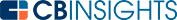# What is the EAR formula?The EAR (effective annual interest rate) formula is a calculation used to determine the rate of interest either earned or paid in a year when adjusted for compounding.

Sometimes referred to as the effective interest rate (EIR) or simply the effective annual rate, the EAR formula allows you to assess how much interest is payable on a loan with compounding interest or how much interest you’ll earn from an investment with compounding interest.

## The importance of the effective annual rate

Several variables can impact return on investment. These variables include:

This applies not only to investments but also to loans. The return on an investment depends on all the variables mentioned above. When a sum compounds, the nominal interest rate and the total number of periods make a significant difference when comparing loans or investments for the same principal amount. To determine the better investment when comparing alternatives, you can use the effective annual rate formula.

## Calculating the effective annual rate

Effective annual rate (EAR) is the real rate of return for any interest paying instrument when considering the effect of compounding over the total time frame. Even if the nominal interest rate and the principal remain the same, the number of periods in which the sum compounds impacts the rate of return. The formula for calculating EAR is:Here, i is the nominal annual interest rate, and n is the number of periods of compounding every year. Even a lower nominal interest rate can result in a higher return when the amount is compounded more times every year compared to another investment that’s compounded fewer times per year. Let’s look at an example.

## EAR in practice

The nominal interest rate does not clearly define the real return on an investment. Instead, you can use EAR to compare two investment opportunities more accurately.

Take two investment opportunities at the same nominal interest rate and principal for a year:

• Investment A - \$10M with an interest rate of 8.25% compounded quarterly
• Investment B - \$10M with an interest rate of 8.25% compounded monthly

Here’s how to calculate the EAR for the investments:\$10M in investment A for a year results in \$10,850,876, and \$10M investment in investment B for a year produces \$10,856,921. That’s a difference of \$6,045 more produced by investment B in a year, making investment B a better investment opportunity.

Sometimes, an investment with a lower interest rate can also be a better investment. Take the scenario below:

• Investment C - \$10M with an interest rate of 10.25% compounded semi-annually
• Investment D - \$10M with an interest rate of 10.2% compounded monthly

Here’s how to calculate the EAR for the investments:As you can see, the EAR for investment D is higher even though the nominal interest rate is less than that of investment C. A \$10M investment in investment D will produce a return of \$177,970 more than the same amount in investment C at a higher nominal interest rate. EAR is the right tool to assess the real returns between two interest-paying instruments.

The effective annual rate is a more accurate measure of the potential return on an investment or the interest payable on a loan with compounding interest. It’s a valuable formula for comparing two loans or investment opportunities to make sound financial decisions.

When using the EAR formula to compare loans, choose the loan with the lower EAR, which means you’ll pay less interest compared to the loan with the higher EAR. When using EAR to compare investment opportunities, the investment with the higher EAR will provide a greater return.

You may also like# www.cbinsights.com